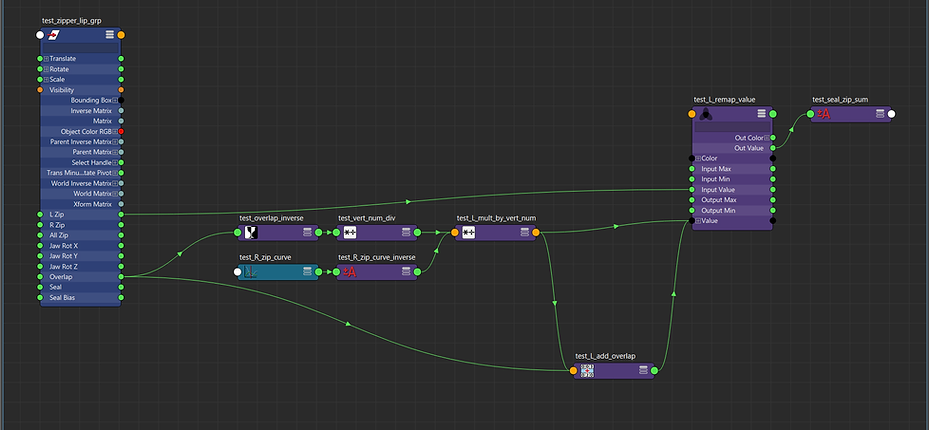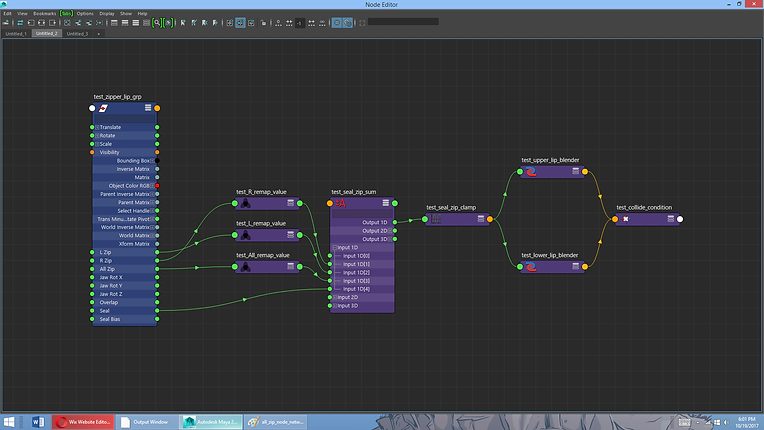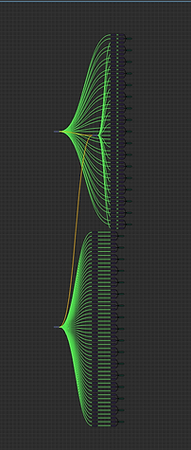top of page#### Zipper Lips Script

##### The second section the script was creating the core node network that would allow the joint chains to zip and seal by blending their rotations. This needed to be relatively easy to understand, so rather than creating a complex node network for each joint, I created one node network and used frame cache nodes to get a unique output for each joint.##### So for the right zipping, we want all of the pairs of joints to blend between the open jaw rotation and the sealed jaw rotation at different rates. Basically we want to set up a series of keyframe animations that look like this.##### So each joint would have it's own curve and the would be set up to go from 0 (0% sealed) to 1 (100% sealed) at different rates, and they would overlap nicely because some of them would be partially sealed at the same time. However setting up this many curves (1 per joint pair would be pretty tedious) and since these curves are pretty uniform we can generate them using a single node network.##### ​##### This difference in the required calculations is the reason the node network is almost identical. The only difference is a plusMinusAverage node which subtracts the vertex number from the number of vertexes.##### This way the node network gives the same output for the last and first vert and all the mirrored pairs of verts (again understanding why this happens requires an understanding of the frameCache node).##### The last step is to add the left zip, right zip, all zip and seal values to determine how much the lips have blended together. Below is that simple node network. The clamp node is needed because putting a value greater than 1 into the blendColor nodes will mess things up so we clamp the sum below 1 (the sum could exceed 1 if you set seal to 1 and left zip and right zip to 1). The blenColor nodes at the right side of the image are the same one from part 1 (we have finally set up the the input for their blender attributes).##### Now the last step is visually the scariest, but only because of the number of nodes, the process is very simple. The "test_upper_lip_blender", "test_lower_lip_blender", and the "test_collide_condition" node will be plugged into the joints. But they will not be plugged in directly. Instead they will plugged into a frameCache node which will allow use to run the node network for all the different values of "vert number". This is the reason we set up animCurves throughout the process. The varyTime value for the frame cache nodes is the vert number the joint is associated with.bottom of page